# Magnetism and Magnetic Properties Important Definitions and Formula

## Magnetism and Magnetic Properties Important Definitions and Formula

MagnetismIMPORTANT DEFINITIONS AND FORMULAE

A. MAGNETS AND THEIR PROPERTIES

1. Magnets:

Magnets are of two types (i) natural magnets and (ii) artificial magnets. Natural magnets are generally irregular in shape and weak in strength. On the other hand artificial magnets have desired shape and desired strength. A bar magnet, a horseshoe magnet, compass needle etc. all are artificial magnets.

2. Properties Of magnets.

(a) Attractive property. A magnet can attract magnetic substance like iron, steel, cobalt etc.

(b) Directive property.

(c) Unlike poles attract and like poles repel.

(d) Magnetic poles exist in pairs.

(e) Force between two magnetic poles obeys inverse square law.

3. Bar magnet and Magnetic Lines of Force:

(a) Magnetic lines of force are imagine lines making closed continuous loops.

(b) Tangent to the magnetic lines of force at any point gives the direction of magnetic field at that point.

(c) Two magnetic field lines cannot interest each other.

(d) Magnetic field lines dilate laterally and contract longitudinally.

(e) Crowded magnetic field lines represent stronger field and vice- versa.

4. Magnetic dipole and Dipole Moment

Two unlike poles of equal strength separated by a small distance make a magnetic dipole. A bar magnet has two magnetic poles known as north pole and south pole each of pole strength m. If the separation between the poles, also known as magnetic length, is then the bar magnet is said to have a magnetic dipole moment.

M = ml

The direction of the magnetic dipole moment is from the south pole of the bar magnet to the north pole of the bar magnet.

5. Magnetic Field Strength at a point due to a Bar Magnet.

B =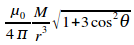Where r is the distance of the point from the centre of the magnet,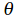is the angle between the magnetic moment vector and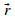.

Special cases:

1. Magnetic field on axial line of a short bar magnet.

B axial =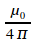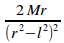Where M = m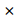2l [m is pole strength].

2. Magnetic field on equatorial line due to a short bar magnet,

B equal =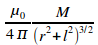6. Torque on a magnetic Dipole in Uniform Magnetic Field.

A magnetic dipole (e.g bar magnet) of dipole moment M when placed in uniform magnetic field B (e.g earth’s magnetic field at a place) experience a torque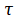= MB sinHereis the angle between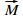and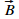.

Vector ally,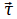=7. Potential Energy of magnetic Dipole in a Uniform Magnetic Field.

U = – MB (cos2– cos1)

8. Electromagnets.

A magnet formed temporarily due to a magnetic field of a current is called electromagnet. Strength of an electromagnet depends upon

1. the number of turns per unit length in a solenoid.

2. Strength of current

3. Nature of the core on which turns are wound.

Material used for making electromagnet must have minimum hysteresis loss. For low volume of magneting field intensity (H) values of magnetic induction () and intensity of magnetisation (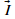) must be high.

9. Permanent Magnets.

Material used for making permanent magnet should have high value of residual magnetism and high value of coercively.

Steel is suitable for making permanent magnets.

B. EARTH’S MAGNETISM

1. Magnetic Field of Earth its Cause.

Earth acts like huge magnet. It’s magnetic field is fairly uniform and its value on the surface of earth is about 10-4 tesla or one gauss. Earth’s magnetic field might be due to:

(i) molten charged metallic fluid in the core of earth.

(ii) due to rotation of earth about its axis.

(iii) due to outer layer of our atmosphere i.e. ionosphere.

2. Magnetic Elements of the Earth’s magnetic Field.

(a) Declination. It is the angle between the magnetic meridian and geographic meridian at a place.

(b) Dip. It is the angle that the intensity of earth’s magnetic field makes with the horizontal at a place. It is represented by the symbol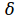. Angle of dip is measured by a dip circle which has to be held in the magnetic meridian of the earth. Angle of dip is 00 at magnetic poles of the earth.

The angle of dip will have more value at northern hemisphere than at southern hemisphere.

(c) Horizontal Component of Earth’s

Magnetic field intensity. It is the component of total intensity of earth’s magnetic field along the horizontal. It is represented by the symbol BH.

The horizontal component BH at a place is related to the total field strength B and the angle of dipat that place as

BH = B cos.

Also if Bv is the vertical component of the earth’s field at that place then

BV = B sin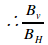= tan.

Also B =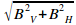3. Magnetic Field of a Bar Magnet and Neutral Points.

(a) N pole of the bar magnet facing magnetic N pole of the earth. Here the neutral point lies on the axial line of the magnet.

Neutral point is that point at which net magnet field, resultant of the magnetic field of the magnet and magnetic field due to earth, is zero.

Here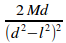= BH

(b) N pole of the bar magnet facing magnetic S pole of the earth. The neutral point N1 and N2 lie on the equator line of the magnet.

Here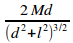= BH

4. Tangent Law.

When a magnetic needle is under the influence of two uniform magnetic field B1 and B2 in mutually perpendicular directions, the magnetic needle will align itself along the resultant field.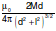If the resultant of B1 and B2 makes an angle with B1 then

tan=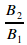or B2 = B1 tan.

C. CLASSIFICATION OF MAGNETIC MATERIALS

1. Atom as a magnetic dipole, 2. Current loop behaves like a magnet 3. Solenoid behaves exactly like a bar magnet, 4. Important terms use in magnetism 5. Classification of magnetic materials, 6. Curie’s law in magnetism.

1. Atom as a Magnetic Dipole.

Each orbit of an electron in an atom acts like a magnetic dipole. Magnetic dipole moment of an orbit of radius r having electron with angular velocity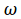. is given by

M = n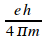M = n (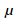0)

Where0 is called Bohr Magneton.0 = 9.2710-24 ampere metre2.

2. Current Loop Behaves Like a Magnet.

The dipole moment of a current-carrying loop is= I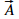Am2

The direction of magnetic moment is from south to north pole of the magnet.

3. Solenoid Behaves Exactly Like a Bar Magnet.

In a bar magnet. Poles are fixed while in solenoid poles can be reversed by reversing the direction of current. Pole strength of a pole in a bar magnet is fixed, but in solenoid, it can be changed by changing the magnitude of current.

4. Important Terms Used in Magnetism.

(i) Magnetic Permeability (). It is the ability of a material to permit magnetic lines of force to pass through it.r =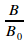=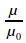Where B0 is no. of magnetic lines of force per unit area in vacuum

B is no. of magnetic lines of force per unit area in the given material medium.

S.I. unit ofis Wb A-1 m -1 or Hm-1 or Tm A-1.

(ii) Magnetising Force or magnetic Intensity

(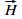). Magnetic intensity represents the degree to which a magnetic material can be magnetized by a magnetic field

H = nl or H =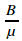Where B is the magnetic induction of flux density.is permeability of the medium

S.I. unit of H is Am-1 or Nm-2 T-1 or Jm-1 Wb-1.

(iii) Intensity of magnetisation (). It is magnetic moment per unit volume of a magnetic material or pole strength per unit area of cross-section of the magnetic material.

I =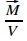or I =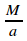.=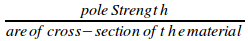S.I. unit of I is Am-1.

(iv) Magnetic Susceptibility:

The magnetic susceptibility (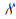) of a specimen is a measure of the ease with which the specimen can be magnetized. It is defined as the ratio of the intensity of magnetization induced in it and the strength of the magnetizing field i.e.=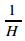5. Classification of magnetic materials:

Different materials are known to behave different materials are known to behave differently in external magnetic field called the magnetizing field and accordingly they. Are categorized as diamagnetic, paramagnetic and ferromagnetic materials.

Diamagnetic materials: (Examples –

1. The vertical component of Earth’s magnetic field at a place is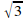times the horizontal component what is the value of angle of dip at this place?

2. Steel is preferred for making permanent magnets whereas soft icon is preferred for making electromagnets. . Give one reason.

3. What is the value of the horizontal component of the earth’s magnetic field at magnetic poles?

4. Give two essential characteristics of a material used for preparing and electromagnet.

5. How does the (i) pole strength and (ii) Magnetic moment of each part of a bar magnet change if it is cut into two equal pieces transverse to its length?

6. How does the (i) pole strength and (ii) Magnetic moment of each part of a bar magnet change if it is cut into two equal pieces along its length?

7. Where on the earth’s surface is the value of angle of dip maximum?

8. Name the physical quantity which has its unit JT-1. Is it scalar or a vector quantity?

9. As shown in Figure a charged particle enters a uniform magnetic field and experiences an upward force as indicated. What is the charge sign on the particle?

10. What are the values of (i) the horizontal component of earth’s magnetic field and (ii) angle of dip. At the magnetic north pole of the earth?

11. Which orientation of magnetic diole in a uniform magnetic field will correspond to its stable equilibrium?

12. What should be the orientation of a magnetic dipole in a uniform magnetic field so that its potential energy is maximum?

13. Write the S.I. unit of (i) magnetic pole strength (ii) magnetic dipole moment of a bar magnet.

14. State the reason why soft iron is used in making electromagnets.

15. What is the value of angle of dip at place on the earth’s surface, where the ratio of the vertical component to the horizontal compoenent of the earth’s magnitude field is 1/?

16. Write the formula for magnetic moment of a current loop.

17. If the ratio of horizontal component of earth’s magnetic field to the resultant magnetic field at a place is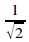, what is the angle of dip at that place?

18. What is the angle of dip at a place where the horizontal and vertical components of the earth’s magnetic field are equal?

19. In which direction would a compass needle align if taken to geographic (i) North and (ii) South pole?

20. How does the intensity of magnetization of a paramagnetic sample vary with temperature?

21. Define paramagnetic substances.

22. Name any two diamagnetic substances.

23. What is reduction factor of galvanometer?

24. State tangent law in magnetism?

25. Horizontal component of Earth’s magnetic field at a place istimes the vertical component. What is the value of angle of dip at this place?

26. Mention two properties of the alloy from which permanent magnets are made.

27. Why do magnetic liens of force prefer to pass through for ferromagnetic substance than through air?

IMPORTANT QUESTIONS

28. What is the direction of magnetic moment vector?

29. What is the SI unit of pole strength?

30. In which position, the potential energy of a magnet in a uniform magnetic field is (i) zero and (ii) maximum.

31. When is a magnet said to be in stable equilibrium in a uniform magnetic field?

32. When does a magnet experience a torque

(i) maximum and (ii) minimum when placed in a uniform magnetic field?

33. who first discovered earth’s magnetism?

34. How much is the approximate distance upto which the earth’s magnetic field extends?

35. What do you mean by the term magnetic axis of the earth?

36. A magnetic needle placed on a piece of cork is floating on the calm surface of a lake in the northern hemisphere. Would the magnetic needle along with the cork move towards the north?

37. Is the earth’s magnetic field inside a closed iron box less or more than that outside it?

38. The earth’s core is known to contain iron, yet geologists do not regard this as a source of earth’s magnetism. Why?

39. What is a neutral point?

40. What is induced magnetism?

41. Define the term intensity of magnetization.

42. Give the order of magnetic moment of an atom.

43. The magnetic susceptibility for small specimen has a small negative value. To which class of magnetic substances, does the specimen belong?

44. What do you mean by the statement that “susceptibility of iron is more than that of copper”?

45. What is curie point?

46. If a toroid uses bismuth for its core, will the field in the core be slightly greater or slightly less than when the core is empty?

47. Is the permeability of a ferromagnetic material independent of the magnetic field? If not, is it more for lower or higher fields?

48. Magnetic field lines are always nearly normal to the surface of a ferromagnetic at every point. Why?

49. Would maximum possible magnetization of a paramagnetic sample be of the same order of magnitude as the magnetization of a ferromagnetic?

50. What is the S.I. unit of (a) magnetic flux (b) magnetic field strength?

51. What is geomagnetic equator?

52. A small magnet is pivoted to move freely in the magnetic meridian. At which place on the earth will the magnet be vertical?

53. What happens if an iron bar magnet is melted? Does it retain its magnetism?

54. Can you think of a magnetic field configuration with three poles?

55. An iron bar magnet is heated to 10000C and then colled in a magnetic field-free space. Will it retain its magnetism?

56. Angle of declination at a place is 180 . How can a magnetic compass be used to find the exact direction of geographical North at that place?

NCERT QUESTIONS

57. What happens if a bar magnet is cut into two pieces (i) transverse to its length (ii) along its length?

58. Must every magnetic field configuration have a north pole and south pole? What about the field due to a toroid?

59. Two identical looking iron bars A and B are given, one of which is definitely known to be magnetized (we do not know which one). How would one ascertain whether or not both are magnetized? If only one is magnetized. How does one ascertain which one? (Use nothing else but the two bars A and B).

60. A vector needs three quantizes for its specification. Name the three independent quantities conventionally use to specify the earth’s magnetic field.

61. The angle of dip at a location in southern India is about 180. Would you except a greater or smaller dip angle in Britain?

62. If you made map of magnetic field lines at Melbourne in Australia, would the lines seem to go into the ground or come out of the ground?

63. In which direction would a compass free to move in the vertical plane point to, if located right on the geomagnetic north or south pole?

64. The earth’s field, it is claimed, roughly approximates the field due to a dipole of magnetic moment 8 1022 JT-1 located at its centre. Check the order of magnitude of this number in some way.

65. Geologists claim that besides the main magnetic N-S poles, there are several local poles on the earth’s surface oriented in different direction. How is such a thing possible at all?

PREVIOUS QUESTIONS

1. Why does a paramagnetic substance display greater magnetization for the same magnetizing field when cooled? How does a diamagnetic substance respond to similar temperature changes?

2. Define the term magnetic inclination and the horizontal component of Earth’s magnetic field at a place. Establish the relationship between the two with the help of a diagram.

3. Define the terms ‘ Magnetic Dip’ and “ magnetic Declination’ with the help relevant diagrams.

4. Write two characteristic properties to distinguish between diamagnetic and paramagnetic materials.

5. Derive and expression for the potential energy of a magnetic dipole of dipole momentin a uniform magnetic field.

6. Explain with the help of diagram the terms (i) magnetic declination and (ii) angle of dip at a given place.

7. Distinguish between diamagnetic and ferromagnetic materials in respect of their (i) intensity of magnetization, (ii) behavior in a non- uniform magnetic field and (iii) susceptibility.

8. Define angle of dip. Deduce the relation connecting angle of dip and horizontal component of earth’s magnetic field at a place.

9. Define the term ‘angle of dip’. What is the value of angle of dip at the magnetic equator? What does it mean?

10. How will you distinguish a diamagnetic substance from a paramagnetic substance in respect of their behavior in a uniform and non-uniform field?

11. A magnetic compass needle of magnetic moment 60 Am2 is placed at a place. The needle points towards the geographical north. Using the data given below, find the value of declination at that place. Horizontal component of earth’s magnetic field = 4010-6 Wb m-2 and torque experienced by the needle = 1.210-3 Nm.

12. Define neutral point. Draw magnetic lines of force when two identical magnets are placed at a finite distance apart with their N-poles facing each other. Locate the neutral point.

13. Define neutral point. Draw magnetic lines of force when two identical magnets are placed at a finite distance apart with their S-poles facing each other. Locate the neutral point.

14. A magnetic needle free to rotate in a horizontal position orients itself with its axis vertical at a certain place on the earth. What are the values of (i) angle of dip and (ii) horizontal components of earth’s field at this place? Where will this place be on earth?

15. Define neutral point. Sketch the lines of magnetic field when a small bar magnet is place with N-pole towards south of the earth. Indicate the position of neutral points.

16. A short bar magnet placed with its axis at 300 to a uniform magnetic field of 0.2 experiences a torque of 0.060 Nm. (i) Calculate the magnetic moment of the magnet and (ii) find out what orientation of the magnet corresponds to its stable equilibrium in the magnetic field.

17. Define the terms: intensity of magnetization and ‘magnetic permeability of a magnetic material’. State two essential characteristics of a magnetic material to be used in preparing a permanent magnet.

18. A magnetic needle free to rotate in a vertical position orients itself with its axis horizontal at a certain place on the earth. What are the values of (i) angle of dip and (ii) horizontal components of the earth’s field at this place? Where will this place be on earth?

19. Define ‘ intensity of magnetization’ of a magnetic material. How does it vary with temperature for a paramagnetic material?

20. A bar magnet of magnetic moment M is aligned parallel to the direction of a uniform magnetic field B. What is the work done, to turn the magnets, so as to align its magnetic moment:

(i) opposite to the field direction and

(ii) normal to the field direction?

21. Define the term ‘angle of dip’. Derive the relation between angle of dip and the resultant magnetic field of earth at a place.

22. A magnetized needle is place 300 with the direction of uniform magnetic field of intensity 310-2T. The torque acting on the needle is 7.210-4J. Calculate the magnetic moment of the needle .

23. A magnetized needle of magnetic moment 4.810-2 J T-1 is placed at 300 with the direction of uniform magnetic field of magnitude 310-2T. Calculate the torque acting on the needle.

24. A magnetized needle of magnetic moment 4.810-2 J T-1 is placed at 300 with the direction of uniform magnetic field. The torque acting on the needle is 7.210-4J. Calculate the magnitude of magnetic field.

25. A magnet makes angular oscillations in a horizontal plane with time periods T1 and T2 at two places, where the horizontal components of earth’s magnetic field are BH and B’H respectively. Deduce and expression to compare the horizontal components at the two places.

26. The susceptibility of a magnetic material is -0.085. Identify the magnetic type of the material. A specimen of this material is kept in a non-uniform magnetic field. Draw the modified field pattern.

27. Distinguish between a diamagnetic substance and a paramagnetic substance stating two points of difference.

28. The susceptibility of a magnetic material is 0.9853. Indentify the type of the magnetic material. Draw the modification of field pattern on keeping a piece of this material in a uniform magnetic field.

29. A coil of 200 turns has cross- Sectional are 900 mm2. It carries a current of 2 ampere. The plane of the coil is perpendicular to a uniform magnetic field of 0.5 T. Calculate (i) the magnetic moment of the coil and (ii) the torque acting on the coil.

30. Define the terms ‘angle of dip’ and ‘ magnetic declination’. The angle of dip at a place in Kerala is 180.Will its value be more or less at a place in Kashmir? Give reason.

31. A magnetic needle suspended freely in a uniform magnetic field experiences a torque but not net force. An iron mail near a bar magnet.

However experiences a force of attraction in addition to torque. Why?

32. Define the terms magnetic inclination and horizontal component of Earth’s magnetic field at a place. Establish the relationship between the two with the help of a diagram.

33. You are given two identical looking bars A and B. One of these is a bar magnet and the other and ordinary piece of iron. Give an experiment to identify which one of the two is a bar magnet. You are not to use any additional materials for the experiment.

34. The vertical component of earth’s magnetic field at a place is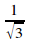times the horizontal component. What is the value of angle of dip at this place?

35. Write any three characteristics, a ferromagnetic substance should posses, if it is to be use to make a permanent magnet. Give one example of such material.

36. State and illustrate Curie’s law in magnetism.

IMPORTANT QUESTION

37. Magnetic field arises due to charges in motion. Can a system have magnetic moment even through its net charge is zero?

38. Magnetic field lines show the direction (at every point), which a small magnetised needle takes up (at the point). Do the magnetic field lines also represent ‘the line of force’ on a moving charged particle at every point?

39. Define magnetic susceptibility. Give its SI unit.

40. What is diamagnetism? Explain.

41. What is paramagnetism? Explain.

42. What is ferromagnetism? Explain.

43. Why do we prefer steel or alnico for making permanent magnets?

44. Give four basic properties of magnets.

45. You are given two identical bar magnets having different dipole moments. How will you select the one having larger dipole moment?

46. Give two points to compare the magnetic properties of steel and soft iron.

47. Does the magnetisation of a paramagnetic salt depend on temperature? Give reason for your answer.

48. Does an iron bar magnet retain its magnetism when melted? Give reason your answer.

49. The earth’s magnetic field varies from point to point in space. Does it also change with time? If so, on what time scale does it change appreciably?

50. The earth’s core is known to contain iron. Yet geologists do not regard this as a source of the earth’s magnetism. Why?

51. The charged currents in the outer conducting regions of the earth’s core are thought to be responsible for earth’s magnetism. What might be the ‘battery’ (i.e the source of energy) to sustain these currents?

52. The earth may have even reversed the direction of its field several times during its history of 4 to 5 billion years. How can geologists know about the earth’s field in such distant past?

53. The earth’s field departs from its dipole shape substantially at large distances (greater than about 30,000 km). What agencies may be responsible for this distortion?

54. Interstellar space has an extremely weak magnetic field of the order of 10-12 T. Can such a weak field be of any significant consequence? Explain.

55. Magnetic force is always normal to the velocity of a charge and therefore does no work. An iron nail held near a magnet, when released , increases its kinetic energy as it moves to cling to the magnet. What agency is responsible for this increase in kinetic energy if not the magnetic field?

56. Many of the figures given in Fig. show magnetic field lines wrongly (thick lines in the figure). Point out what is wrong with them. Some of them may describe electrostatic field lines correctly. Point out which ones.

57. Adjoining figure shows a small magnetised needle P placed at a point O. The arrow shows the direction of its magnetic moment. The other arrows show different positions moment) of another identical magnetised needle Q.

(a) In which configuration is the system in

(i) stable, and (ii) unstable equilibrium?

(c) Which configuration corresponds to the lowest potential energy among all the configurations shown?

58. Magnetic field lines show the direction (at every point) along which a small magnetised needle aligns (at that point). Do the magnetic field lines also represent the ‘ lines of force’ on a moving charged particle at every point?

59. Magnetic field lines can be entirely confined within the core of a toroid, but not within a straight solenoid. Why?

60. If magnetic monopoles existed, how would the Gauss’ law of magnetism be modified?

61. Does a bar magnet exert a torque on itself due to its own field? Does one element of a current carrying wire exert a force on another element of the same wire?

62. Magnetic field arises due to charges in motion. Can a system have magnetic moments even though its net charge is zero?

63. Why does a paramagnetic sample display greater magnetisation (for the same magnetising field) when cooled?

64. Why is diamagnetism, in contrast, almost independent of temperature?

65. If a toroid uses bismuth for its core, will the field in the core be (slightly) greater or ( slightly) less than when the core is empty?

66. Is the permeability of a ferromagnetic material independent of the magnetic field? If not, is it more for lower or higher fields?

67. Magnetic field lines are always nearly normal to the surface of a ferromagnet at every point (This fact is analogous to the static electric field lines being normal to the surface of a conductor at every point). Why?

68. Would the maximum possible magnetisation of a paramagnetic sample be of the same order of magnitude as the magnetisation of a ferromagnet?

69. Explain qualitatively on the basis of domain picture the irreversibility in the magnetisation curve of a ferromagnet.

70. The hysteresis loop of a soft iron piece has a much smaller area than that of a carbon steel piece. If the material is to go through repeated cycles of magnetization, which piece will dissipate greater heat energy?

71. what kind of ferromagnetic material is used for casting magnetic tapes in a cassette player. Or for building ‘memory stores’ in a modern computer?

72. A certain region of space is to be shielded from magnetic fields. Suggest a method.

73. A short bar magnet placed with its axis at 300 with a uniform external magnetic field of with a uniform external magnetic field of 0.25 T experiences a torque of magnitude equal to 4..510-2 J. What is the magnitude of magnetic moment of the magnet?

74. A magnetic needle free to rotate in a vertical plane parallel to the magnetic meridian has its north tip pointing down at 220 with the horizontal. The horizontal component of the earth’s magnetic field at the place is known to be 0.35 G. Determine the magnitude of the earth’s magnetic field at the place.

75. At a certain location in Africa, a compass points 120 west of the geographic north. The north tip of the magnetic needle of a dip circle placed in the plane of magnetic meridian points 600 above the horizontal. The horizontal component of the earth’s field is measured to be 0.16 G. Specify the direction and magnitude of the earth’s field at the location.

76. Given the magnetic needle has magnetic moment 6.710-2 Am2 and moment of inertia I = 7.510-6 kgm2. It performs 10 complete oscillation in 6.70 s. What is the magnitude of the magnetic field?

77. The earth’s magnetic field at the equator is approximately 0.4G. Estimate the earth’s dipole moment.

78. In the magnetic meridian of a certain place, the horizontal component of the earth’s magnetic field is 0.26G and the dip angle is 600. What is the magnetic field of the earth at this location?

79. What is the magnitude of the equatorial and axial fields due to a bar magnet of length 5.0 cm at a distance of 50 cm from its midpoint? The magnetic moment of the bar magnet is 0.40 Am2.